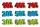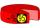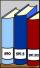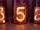Tickets

On Monday, 33 tickets were sold. Every next day, twice as many as the previous day. How many tickets were sold on Friday and how many totally from Monday to Friday?

Result

l5 =  528
s =  1023

Solution:Leave us a comment of this math problem and its solution (i.e. if it is still somewhat unclear...):Be the first to comment!Next similar math problems:

1. GlovesI have a box with two hundred pieces of gloves in total, split into ten parcels of twenty pieces, and I sell three parcels. What percent of the total amount I sold?
2. Down syndromeDown syndrome is one of the serious diseases caused by a gene mutation. Down syndrome occurs in approximately every 550-born child. Express the incidence of Down's syndrome in newborns at per mille.
3. The percentages in practiceIf every tenth apple on the tree is rotten it can be expressed by percentages: 10% of the apples on the tree is rotten. Tell percent using the following information: a. in June rained 6 days b, increase worker pay 500 euros to 50 euros c, grabbed 21 fro
4. Base, percents, valueBase is 344084 which is 100 %. How many percent is 384177?
5. Sales offGoods is worth € 70 and the price of goods fell two weeks in a row by 10%. How many % decreased overall?
6. Combine / add termCombine like terms 4c+c-7c
7. Double percentWhat is 80% of 60% of 2800?
8. The squareThe square root of 25 times the square root of 81 is what number?
9. PercentagesExpressed as a percentage:
10. Watching TVOne evening 2/3 students watch TV. Of those students, 3/8 watched a reality show. Of the students that watched the show, 1/4 of them recorded it. What fraction of the students watched and recorded reality tv.
11. Decimal expansionCalculate: 2 . 1 + 0 . 10 + 7 . 10000 + 4 . 1000 + 6 . 100 + 0 . 100000 =
12. Simplify 2Simplify expression: 5ab-7+3ba-9
13. TVsProduction of television sets increased from 3,500 units to 4,200 units. Calculate the percentage of production increase.What will be hexadecimal number 303 as decimal number?Identify unknown number which 1/5 is 40 greater than one tenth of that number.What number is 20 % smaller than the number 198?148 is the total number of employees. The conference was attended by 22 employees. How much is it in percent?